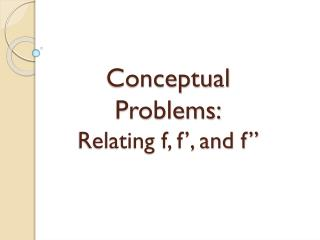DownloadDownload PresentationConceptual Problems: Relating f, f’, and f”

# Conceptual Problems: Relating f, f’, and f”

Télécharger la présentation## Conceptual Problems: Relating f, f’, and f”

- - - - - - - - - - - - - - - - - - - - - - - - - - - E N D - - - - - - - - - - - - - - - - - - - - - - - - - - -
##### Presentation Transcript

1. Conceptual Problems:Relating f, f’, and f”

2. Problem A

3. Problem B

4. Conceptual Problems • Inability to see derivative as a function, only a value • Derivative is object but not as an operation • Derivative vs. Differentiation vs. “Finding the derivative” • Derivative at a point predicting local behavior • Symbolically • Graphically • From a table • Even after understanding the relationship between f and f”, making the connection between f’ and f” • Notation & Meaning of Notation • Writes f’(x)=3 when they mean f’(4)=3 • Translating from a forward proficiency f -> f’-> f” to “given multiple representations, how can we relate them?”

5. Skill vs. Understanding • If Problem A was given in terms of the polynomials, far fewer students would have difficulty identifying which is the derivative and second derivative. An important part of understanding the concept of derivative is multiple representations. (graphs, tables, etc.) • If the skill of finding the derivative at a given point is over-stressed, the understanding of the derivative as a function may not materialize.

6. Language • General use of “it”; “it is concave up because it is positive” vs. “the graph of f is concave up on the given interval because its second derivative is positive on that interval” • The issue is primarily learning to attach the correct characteristic to a specific noun, and then giving the proper implication • Early in the Calc curriculum, it needs to be made clear what the proper communication of an answer looks like • Students need to be instructed on the difference between meaning and “meaning by association” • f” is concave up because it is positive

7. Ability to Transport Knowledge • Associating the rules of the common specific examples to general rules for the given operation (improper generalization) • Taking the derivative eliminates one “hump” of a graph; then trying to apply this rule to a sine graph • Even if there is a strong understanding of the rules, applying that rule to a alternate situation can still prove difficult. • After achieving an understanding of Problem A with the prompts; f, f’, f”, not being able to solve the same problem with the prompts; position, velocity, and acceleration.

8. Practice • Given a function; find the first and second derivatives in symbolic form OR Given a function; graph the function along with the graphs of the first and second derivatives • Given the graph of a function, graph the first and second derivatives • Problem A • Given the graph of the first or second derivative and enough additional information; find an approximate graph of the original function ORGiven the first or second derivative in symbolic form along with enough additional information; find the symbolic form of the original function • Problem B • Do a problem similar to Problem B from information in a table